﻿ 城市群扩容是否有利于产业升级《上海财经大学学报》
2021第23卷第3期图 1 城市群扩容的传导机制
(一) 要素优化配置效应

(二) 市场规模扩大效应

(三) 技术创新效应

(四) 政府合作效应

${D}_{jt}$

${Y}_{jt}$

${\alpha }_{jt}{D}_{jt}$

${Y}_{jt}^{N}$

${Y}_{jt}^{N}$

 $\begin{array}{l} {Y}_{jt}={\alpha }_{jt}{D}_{jt}+{Y}_{jt}^{N} ={\alpha }_{jt}{D}_{jt}+({\delta }_{t}+{\theta }_{t}{Z}_{j}+{\lambda }_{t}{\mu }_{j}+{\varepsilon }_{jt}) \end{array}$ (1)

${\delta }_{t}$

${Z}_{j}$
$(r\times 1)$

${\theta }_{t}$
$(1\times r)$

${\mu }_{j}$

${\lambda }_{t}$

${\varepsilon }_{jt}$

${Y}_{jt}^{N}$

 ${\widehat{\alpha }}_{1t}={Y}_{1t}-\sum\nolimits_{j\geqslant 2}{w}_{j}{Y}_{jt}$ (2)

${\lambda }_{t}{\mu }_{j}={\phi }_{j}$
，则式（1）简化为传统的固定效应模型，但固定效应模型只允许存在未观察到且不随时间变化的异质性，而SCM使用的因子模型则允许未观察到的混杂因素随时间变化，即在控制可观察值之后，已处理和未处理单元之间可以存在非平行趋势。具体来说，假设共有（
$1+J$
）个地区，其中第1个地区为受到扩容冲击的城市，而其余
$J$

$T$

${T}_{0}$

${Y}_{j}$
$(T\times 1)$

$j$

${Y}_{0}$
$(T\times J)$

$W$
$(J\times 1)$

$({w}_{2},{w}_{3},\cdots ,{w}_{J+1}){'}$
，且满足
$\sum\nolimits_{j=2}^{J+1}{w}_{j}=1$
${w}_{j}\geqslant 0,\forall j\in \{2,\cdots ,J+1\}$
。构造对照组结果变量的加权平均值
${Y}_{0}W$
，将结果分为预处理向量
${ Y_j}$

${\vec Y_j}$
，并令X代表k个预处理特征（即预测变量）均值构成的向量，其中X包括协变量Z以及结果变量部分预处理向量
$\mathop Y\limits^{\leftharpoonup}$
M个线性组合，因此
$k=r+M$
。类似地，令
${X}_{0}$
$(k\times J)$

$V$
$(k\times k)$

$Y$
$X$

$W$
$V$
。对于给定的
$V$
，选择
$W$

${||{X}_{1}{-X}_{0}W||}_{V}$

$V$

$V$

$\sum\nolimits_{t=1}^{{T}_{0}}{\lambda }_{t}{'}{\lambda }_{t}$

${\widehat{\alpha }}_{1}$

${\varepsilon }_{jt}$

(二) 变量说明

1. 产业升级变量。中国整体工业化进程已经进入中后期，在今后较长一段时间内，工业化的重点不仅是推动三次产业结构的变化，更在于通过工业化提升经济发展质量和竞争力，而技术进步正是实现这种攀升的基本动力。因此，本文借鉴周茂等（2018）的方法，结合各地区制造业内部产业结构和细分产业的技术复杂度，从技术进步的角度刻画产业升级。因为无论企业采用哪种升级策略，无论企业在产品或产业价值链上发生怎样的变化，最终都会在制造业内部使产业结构和细分产业的技术复杂度得到体现。为了计算产业升级指标，本文首先参照Hausmann等（2007）的方法计算行业内HS6位产品层面的技术复杂度，计算公式如下：

 ${Prody}_{k}=\displaystyle\sum \frac{({Export}_{mk}/{Export}_{m})\times {Y}_{m}}{\displaystyle\sum\nolimits_{m}{Export}_{mk}/{Export}_{m}}$ (3)

${Prody}_{k}$

$k$

${Export}_{mk}/{Export}_{m}$

$m$

$k$

${Y}_{m}$

$m$

${soph}_{ct}$
）作为产业升级指标。与常用的出口复杂度相比，地区技术复杂度的优势在于通过测度一个地区制造业生产结构的升级而不仅是出口结构的升级，可以更为方便地识别地区内产业结构变化的影响。具体计算方法如下：

 ${soph}_{ct}=\frac{\displaystyle\sum\nolimits_{i}{Output}_{i,c,t}\times {Prody}_{i,1997}}{\displaystyle\sum\nolimits_{i}{Output}_{i,c,t}}$ (4)

${Prody}_{i,1997}$

$i$

2. 控制变量。根据相关研究，本文将影响产业升级的主要因素设定为经济发展水平（pergdp）、城市化率（urban）、基础设施水平（infra）、政府规模（gov）、金融发展水平（finan）、人力资本水平（human）和人口密度（density）。其中，经济发展水平是一个地区产业发展的基础，对地区产业结构产生巨大影响，本文采用人均地区生产总值（万元/人）表示；城市化率反映了产业所需的实物资本和人力资本向城市积累的路径，为产业发展提供了大量建成区土地和劳动力，考虑到数据的可获得性，本文使用第三产业增加值占GDP比重（%）衡量；金融发展水平采用地区年末金融机构贷款余额与地区生产总值的比值（%）来表示；基础设施对产业发展具有重要作用，采用固定资产投资占GDP的比重（%）来计算；政府规模反映了地区政府的调控水平，用地区财政支出占地区生产总值的比重（%）来表示；人力资本水平用中等学校在校人数占总人数的比例（%）来表示；人口密度采用每平方公里人口数（人/平方公里）来度量。

3. 样本选择与数据说明。县域产业复杂度的计算主要基于中国工业企业数据库的微观数据，由于工业企业数据库存在企业数据误报情况，使用该数据库估算区域产业复杂度和进行实证研究前需要清理异常值。参照Brandt等（2012）和聂辉华等（2012），本文做如下处理：剔除总资产、固定资产、中间投入品与工业增加值等主要缺失变量或者小于0的企业样本；为了保持非国有企业与国有企业的可比性，本文选择保留“规模以上”标准的观测值，即去除企业雇员人数小于30人的企业样本。

 变量名称 单位 样本量 均值 标准差 最小值 最大值 地区技术复杂度（szong） 千美元 2744 12.607 20.170 4.543 20.245 经济发展水平（lnpergdp） （万元/人）的对数 2744 9.322 0.874 7.415 11.716 城市化率（urban） % 2744 0.352 0.068 0.182 0.577 基础设施水平（infra） % 2744 0.419 0.327 0.005 1.027 政府规模（gov） % 2744 0.143 0.074 0.036 0.522 金融发展水平（finan） % 2744 0.674 0.358 0.167 1.643 人力资本水平（human） % 2744 0.060 0.014 0.023 0.113 人口密度（lndensity） （人/平方公里）的对数 2744 3.135 0.738 1.151 4.865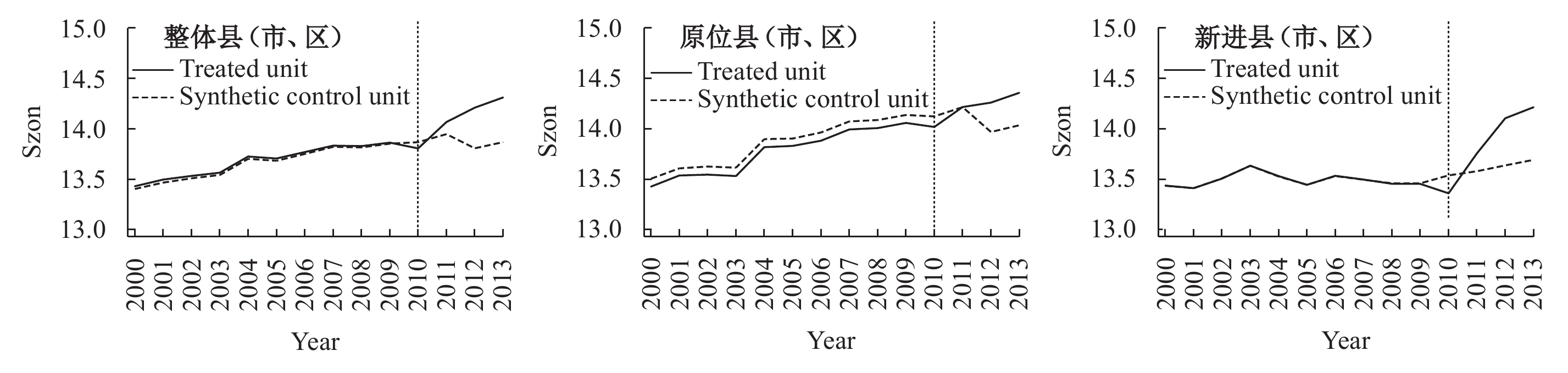图 2 县级行政区实际与合成的产业升级路径对比

 预测变量 整体 原位 新进 真实值 合成值 真实值 合成值 真实值 合成值 经济发展水平 10.157 9.132 10.343 9.210 9.599 9.083 城市化率 0.349 0.357 0.357 0.359 0.332 0.359 基础设施水平 0.281 0.322 0.259 0.325 0.326 0.325 政府规模 0.080 0.122 0.074 0.123 0.093 0.124 金融发展水平 0.776 0.664 0.797 0.670 0.730 0.649 人力资本水平 0.057 0.357 0.056 0.062 0.059 0.063 人口密度 3.018 3.206 2.895 3.262 3.233 3.165
(二) 安慰剂检验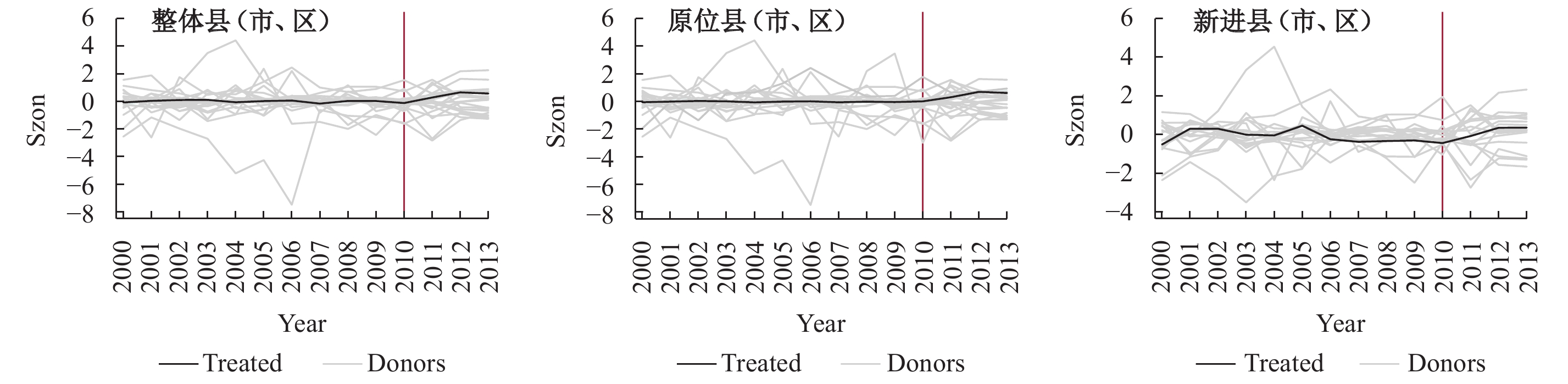图 3 安慰剂检验——产业技术复杂度差值分布
(三) 稳健性检验

1. 更换因变量度量指标。在基准回归中，本文在对地区产业结构进行度量时采用的是不同产业的规模数据，那么前文结论是否是由于计算方法的特殊性造成的？为此，本文采用从业人数和实用资本代替工业总产出赋予县域层面新的产业结构权重，对因变量进行重新计算。检验结果显示，三类县（市、区）在扩容发生后均表现出产业升级现象，即因变量产业结构权重改变后基准结果依旧稳健。

2. 更换对照组。长三角地区行政区划调整频繁，这些因素对该区域的产业升级可能产生影响。为了得到更加“干净”的扩容效应，本文对所选的合成样本进行调整和控制：（1）长三角地区撤地设市、撤县设区、撤县设市等行政区划调整在1998−2005年间较为频繁，1997年长三角新增15个城市（上海、无锡、宁波、舟山、苏州、扬州、杭州、绍兴、南京、南通、常州、泰州、湖州、嘉兴、镇江），以及中国在2001年加入WTO等都会对产业升级造成影响（周茂等，2016，2018）。因此，本节对2000–2005年的样本进行剔除，仅观察2006–2013年（共8年）的样本合成效果。检验发现，城市群扩容促进产业升级的基准结论依旧稳健。（2）2000–2013年长三角进行了两次主要的扩容，一次是本文采用的政策节点即2010年，另一次发生在2013年协调会第十三次会议上，增补了芜湖、连云港、徐州、滁州、淮南、丽水、宿迁、温州8个城市。为了排除样本期内的第二次扩容对基准结论可能造成的干扰，本文剔除第二次扩容的城市所含县（市、区）后重新进行合成，结果依旧显著。

3. 变换估计方法。考虑到对照组和处理组县级行政区差异较大，我们进一步采用基于倾向得分匹配的双重差分法（PSM-DID）进行检验，表3为回归结果。总体来看，扩容促进了整体县（市、区）的产业升级，原位县（市、区）的促进效果在5%的水平上显著。而新进县（市、区）的结果并不显著且结果为负，可能的原因是新进区域多在安徽的皖江经济带和合肥经济圈，经济发展水平相对较低，尽管加入长三角城市圈后能够享受到更大范围的规模经济和技术溢出，从而带动产业升级，但是新进县（市、区）的生产要素向原位县（市、区）转移，从而不利于产业升级。为了验证这个推测，我们对在其他经济圈中的对照组县（市、区）进行剔除并重新进行PSM-DID估计，发现剔除后的新进县（市、区）的估计结果为正，验证了基准结论的稳健性。

 政策冲击前对照组 政策冲击前处理组 处理组与对照组 的差值 政策冲击后对照组 政策冲击后处理组 处理组与对照组的差值 双重差分值 整体县（市、区） 13.809 13.609 −0.201**（−2.37） 13.883 14.022 0.138（1.03） 0.339**（2.13） 原位县（市、区） 14.191 13.664 −0.527***（−5.20） 14.046 14.090 0.044（0.26） 0.571***（2.92） 新进县（市、区） 12.976 13.483 0.507***（4.48） 13.640 13.878 0.238（1.36） −0.269（1.29） 注：括号内为t统计值，***、**、*分别代表1%、5%和10%的显著性水平，下同。
(四) 异质性检验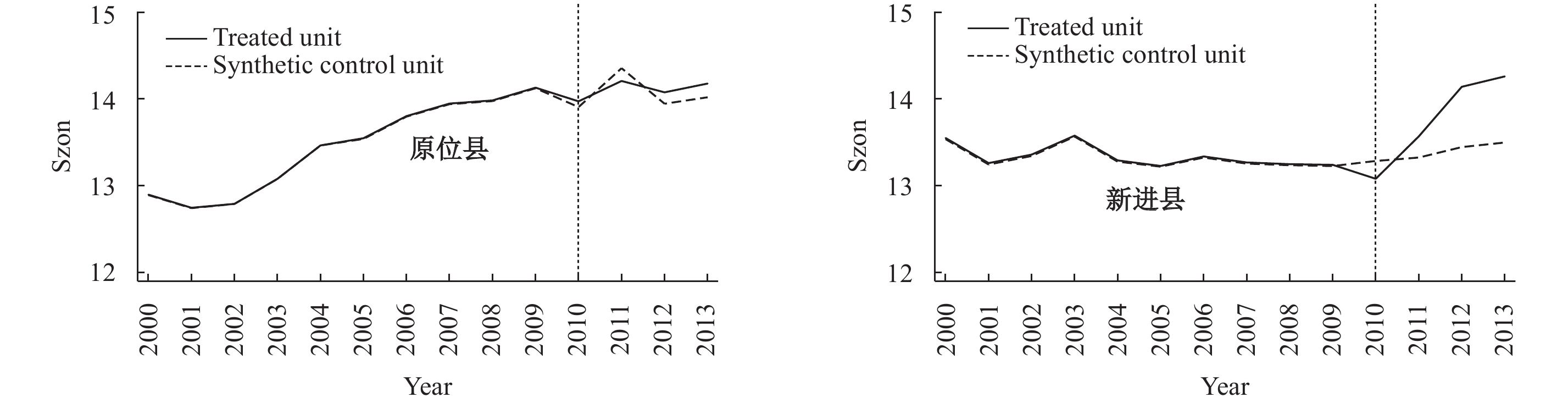图 4 县实际与合成的产业升级路径对比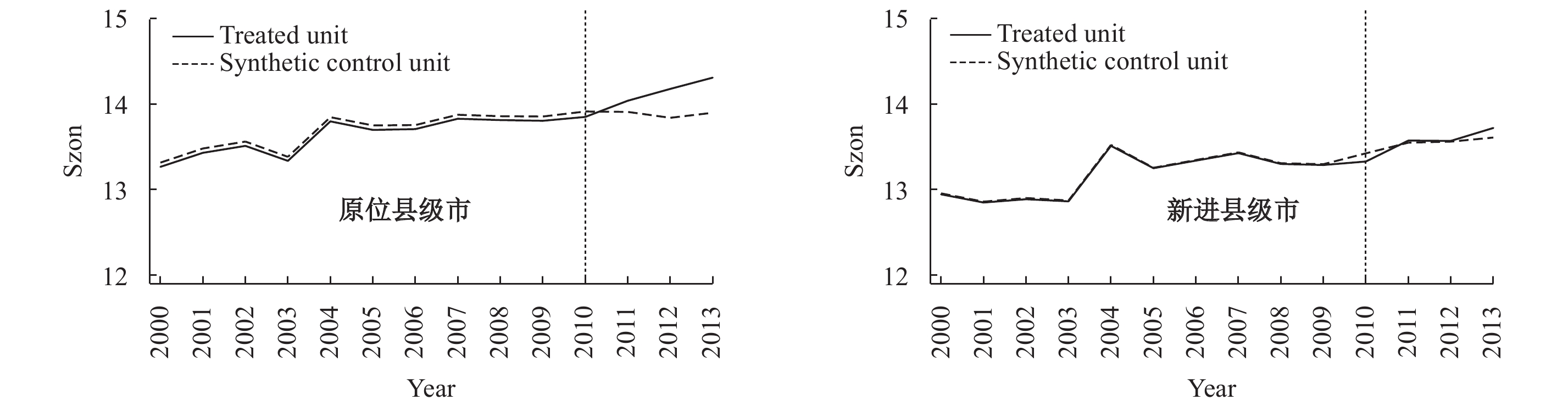图 5 县级市实际与合成的产业升级路径对比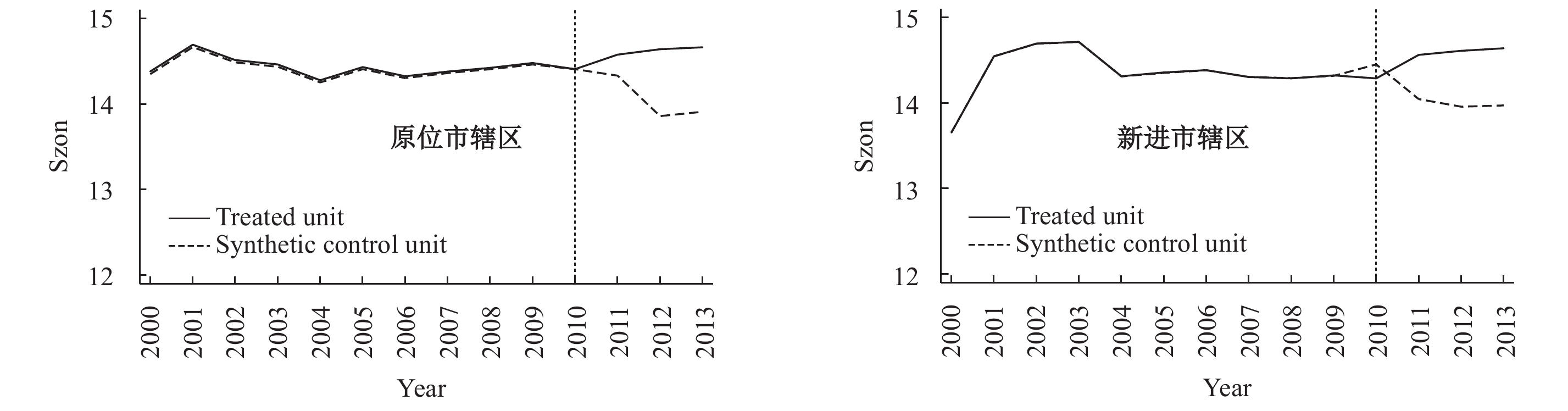图 6 市辖区实际与合成的产业升级路径对比

${Output}_{i,c,t}$
${Prody}_{i,1997}$
${X}_{i,c,t}$
${P}_{i,97}$

 \begin{aligned} \Delta Sop{h_{c,t}} = &\Delta \left( {\sum\nolimits_i {{P_{i,97}}} \frac{{{X_{i,c,t}}}}{{{X_{c,t}}}}} \right)\\ & = \sum\nolimits_{i \in {I^s}} {{P_{i,97}}} \left( {\frac{{{X_{i,c,t}}}}{{{X_{c,t}}}} - \frac{{{X_{i,c,t - 1}}}}{{{X_{c,t - 1}}}}} \right) + \left( {\sum\nolimits_{i \in {I^{entry}}} {{P_{i,97}}} \frac{{{X_{i,c,t}}}}{{{X_{c,t}}}} - \sum\nolimits_{i \in {I^{exit}}} {{P_{i,97}}} \frac{{{X_{i,c,t - 1}}}}{{{X_{c,t}} - 1}}} \right)\\ & = \underbrace {\sum\nolimits_{i \in {I^s}} {{P_{i,97}}} \left( {\frac{{{X_{i,c,t}}}}{{{X_{c,t}}}} - \frac{{{X_{i,c,t}}}}{{{X_{c,t - 1}}}}} \right)}_{\text{县（市、区）间资源再配置}} + \underbrace {\sum\nolimits_{i \in {I^s}} {{P_{i,97}}} \left( {\frac{{{X_{i,c,t}}}}{{{X_{c,t}} - 1}} - \frac{{{X_{i,c,t - 1}}}}{{{X_{c,t - 1}}}}} \right) + \Delta S_{c,t}^{ee}}_{\text{县（市、区）内产业间资源再配置}}\\ & = \Delta S_{c,t}^{inter} + \Delta S_{c,t}^{intra} + \Delta S_{c,t}^{ee} \end{aligned} (5)

$\Delta {Soph}_{c,t}$
）和县（市、区）间的产出变化（
$\Delta {S}_{c,t}^{inter}$
）、县（市、区）内每个产业的产出变化（
$\Delta {S}_{c,t}^{intra}$
）以及误差项（
$\Delta {S}_{c,t}^{ee}$
）共同决定，其中前两项都是建立在持续生存的产业基础上，误差项代表产业的进入与退出。根据以上分解结果，将三个分解变量分别替代原来的被解释变量进行回归，表4展示了整体县（市、区）的资源再配置效应。从表4可以发现，长三角扩容对产业升级的影响主要来自不同县（市、区）间再配置，即县（市、区）间的资源重新配置提升了要素的配置效率。这一结果反映了城市群扩容会通过产业结构调整和要素优化配置推动产业升级。

 被解释变量：县（市、区） 产业升级 （1）不同县（市、区）间再配置 （2）县（市、区）内持续生存产业间再配置 （3）产业进入退出 $\Delta {S}_{c,t}^{inter}$ $\Delta {S}_{c,t}^{intra}$ $\Delta {S}_{c,t}^{ee}$ $\Delta {did}_{ct}$ 14.320* −14.306* 0.886 （1.76） （−1.78） （0.60） R2 0.004 0.005 0.020 观测值 2412 2412 2412
(二) 中介路径检验

 ${S\!oph{'}}_{ct}={c}{did}_{ct}+\theta {X}_{ct}+{\sigma }_{c}+{\sigma }_{t}+{\varepsilon }_{ct}$ (6)
 ${MV}_{ct}=a{did}_{ct}+\theta {X}_{ct}+{\sigma }_{c}+{\sigma }_{t}+{\varepsilon }_{ct}$ (7)
 ${S\!oph{'}}_{ct}=c{'}{did}_{ct}+{b}{MV}_{ct}+\theta {X}_{ct}+{\sigma }_{c}+{\sigma }_{t}+{\varepsilon }_{ct}$ (8)

${Soph{'}}_{ct}$

${MV}_{ct}$

${X}_{ct}$

${did}_{ct}$

$c$

${did}_{ct}$

$a$

${MV}_{ct}$

$b$

$ab$

${did}_{ct}$

$c{'}$

${c}={c}^{{'}}+ab$

$a{\text{、}}b$

$a$

$b$

${H}_{0}:ab=0$

${c}^{{'}}$

${c}^{{'}}$

$ab$
${c}^{{'}}$

1. 要素流动指标：参考王必达和苏婧（2020）的研究，以地区在岗职工平均人数和工业企业数作为劳动要素和资本要素的代理变量。考虑到要素流动突出的是流量，所以这里用职工增长人数代表劳动要素流动，企业增长数代表资本要素流动。

2. 市场分割指标：借鉴丁焕峰等（2020）的研究，选取要素层面的劳动参工率和市场层面的市场活跃度两个变量来衡量市场分割削弱。其中，劳动参工率采用单位从业人口占总人口比重（%）来衡量地区劳动人口的数量及素质；市场活跃度以居民消费规模来代表，采用实际人均社会消费品零售总额（元/人）的对数来衡量。

3. 政府竞争指标：参考彭洋等（2019）的研究，选取税收负担与政府补贴两项来表示政府竞争对市场的扭曲。其中，税收负担用企业所得税与利润总额的比重（%）来衡量，政府补贴用企业补贴收入与工业总产值的比值（%）来衡量。

4. 技术创新指标：采用实际财政科技支出（万元）的对数来表示政府对创新的支持，选取专利权申请成功率（%）和增长率（%）作为研发创新外溢效应的另一指标。

 表5a （1） ${S\!oph{'}}_{ct}$ （2）职工增长人数 （3）企业增长数 （4）劳动参工率 （5）市场活跃度 ${did}_{ct}$ 0.231*** 0.228*** −0.012 0.022** 0.102*** （2.90） （4.75） （−0.24） （2.24） （3.41） R2 0.178 0.131 0.487 0.583 0.884 观测值 3072 2680 1915 3070 3057 表5b ${S\!oph{'}}_{ct}$ ${S\!oph{'}}_{ct}$ ${S\!oph{'}}_{ct}$ ${S\!oph{'}}_{ct}$ ${did}_{ct}$ 0.154**（1.99） 0.121**（2.27） 0.223**（2.82） 0.220***（2.77） ${MV}_{ct}$ 0.180（1.13） 0.113*（1.71） 0.362（1.21） 0.088（1.13） R2 0.019 0.020 0.111 0.162 观测值 2680 1915 3070 3057

 表6a （1）税收负担 （2）政府补贴 （3）政府科技支出 （4）专利增长率 （5）专利申请成功率 ${did}_{ct}$ 0.041 0.001** 0.479*** 0.116*** 0.073*** （0.72） （1.98） （4.09） （3.64） （2.93） R2 0.004 0.084 0.556 0.005 0.053 观测值 2880 2688 2109 2870 3061 表6b ${S\!oph{'}}_{ct}$ ${S\!oph{'}}_{ct}$ ${S\!oph{'}}_{ct}$ ${S\!oph{'}}_{ct}$ ${S\!oph{'}}_{ct}$ ${did}_{ct}$ 0.242***（2.79） 0.314***（3.19） 0.138***（2.42） 0.215***（2.84） 0.230***（2.88） ${MV}_{ct}$ 0.002（0.95） 5.057（1.25） 0.041*（1.76） 0.036**（2.40） 0.018（0.35） R2 0.118 0.125 0.100 0.155 0.203 观测值 2880 2688 2109 2870 3061

 被解释变量 解释变量 中介变量 总效应 中介效应 中介效应量 ${S\!oph{'}}_{ct}$ ${did}_{ct}$ ${MV}_{ct}$ $\mathrm{c}$ $ab$ $ab/c$ 产业升级 长三角城市群扩容 职工增长人数 0.231 0.079 34.32% 企业增长数 0.231 0.153 66.38% 市场活跃度 0.231 0.053 23.08% 政府科技支出 0.231 0.095 41.13% 专利增长率 0.231 0.025 10.85% 专利申请成功率 0.231 0.017 7.3%

2021年3月，十三届全国人大第四次会议表决通过了《中华人民共和国国民经济和社会发展第十四个五年规划和2035年远景目标纲要》，其核心是加快构建以国内大循环为主体、国内国际双循环相互促进的新发展格局。促进国内大循环，必须打破国内市场上存在的各种显性与隐性要素流通障碍，通过促进要素在国内的自由流动来塑造大国的统一大市场。长三角经过40多年的发展，已经成为国际大循环的一个支点，深深地融入了世界；同时长三角也是科技创新、高端制造、高端服务和高端消费的所在地，对构建国内大循环至关重要。

① 与周茂等（2016）一致，本文将行业的技术复杂度固定在1997年，主要是为了克服内生性问题，同时排除样本期内产业结构内生变化的干扰。

② 本文把新进入企业定义为第一次出现在数据库中的企业，退出企业为上一年出现在数据库中但从下一年开始不再出现的企业。

  陈云贤. 中国特色社会主义市场经济: 有为政府+有效市场[J].经济研究,2019(1).  丁焕峰, 孙小哲, 刘小勇. 区域扩容能促进新进地区的经济增长吗? ——以珠三角城市群为例的合成控制法分析[J].南方经济,2020(6).  简泽, 谭利萍, 吕大国, 等. 市场竞争的创造性、破坏性与技术升级[J].中国工业经济,2017(5).  李培鑫, 张学良. 长三角空间结构特征及空间一体化发展研究[J].安徽大学学报（哲学社会科学版）,2019(2).  林毅夫, 巫和懋, 邢亦青. “潮涌现象”与产能过剩的形成机制[J].经济研究,2010(10).  刘明宇, 芮明杰. 全球化背景下中国现代产业体系的构建模式研究[J].中国工业经济,2009(5).  刘乃全, 吴友. 长三角扩容能促进区域经济共同增长吗[J].中国工业经济,2017(6).  刘雅媛, 张学良. “长江三角洲”概念的演化与泛化——基于近代以来区域经济格局的研究[J].财经研究,2020(4).  刘志彪. 区域一体化发展的再思考——兼论促进长三角地区一体化发展的政策与手段[J].南京师大学报（社会科学版）,2014(6).  莫长炜, 龙小宁. 产业集群、技术外溢与企业创新绩效[J].厦门大学学报（哲学社会科学版）,2018(1).  聂辉华, 江艇, 杨汝岱. 中国工业企业数据库的使用现状和潜在问题[J].世界经济,2012(5).  彭洋, 许明, 卢娟. 区域一体化对僵尸企业的影响——以撤县设区为例[J].经济科学,2019(6).  王必达, 苏婧. 要素自由流动能实现区域协调发展吗——基于“协调性集聚”的理论假说与实证检验[J].财贸经济,2020(4).  温忠麟, 叶宝娟. 中介效应分析: 方法和模型发展[J].心理科学进展,2014(5).  吴福象, 刘志彪. 城市化群落驱动经济增长的机制研究——来自长三角16个城市的经验证据[J].经济研究,2008(11).  徐康宁, 冯伟. 基于本土市场规模的内生化产业升级: 技术创新的第三条道路[J].中国工业经济,2010(11).  徐现祥, 李郇. 市场一体化与区域协调发展[J].经济研究,2005(12).  许政, 陈钊, 陆铭. 中国城市体系的“中心—外围模式”[J].世界经济,2010(7).  叶静怡, 林佳, 姜蕴璐. 知识溢出、距离与创新——基于长三角城市群的实证分析[J].世界经济文汇,2016(3).  尤济红, 陈喜强. 区域一体化合作是否导致污染转移——来自长三角城市群扩容的证据[J].中国人口·资源与环境,2019(6).  张学良, 李培鑫, 李丽霞. 政府合作、市场整合与城市群经济绩效——基于长三角城市经济协调会的实证检验[J].经济学（季刊）,2017(4).  张学良, 陆铭, 潘英丽. 空间的聚集: 中国的城市群与都市圈发展[M]. 上海: 格致出版社, 上海人民出版社, 2020.  赵领娣, 徐乐. 基于长三角扩容准自然实验的区域一体化水污染效应研究[J].中国人口·资源与环境,2019(3).  周黎安. 晋升博弈中政府官员的激励与合作——兼论我国地方保护主义和重复建设问题长期存在的原因[J].经济研究,2004(6).  周茂, 陆毅, 杜艳, 等. 开发区设立与地区制造业升级[J].中国工业经济,2018(3).  周茂, 陆毅, 符大海. 贸易自由化与中国产业升级: 事实与机制[J].世界经济,2016(10).  Abadie A, Diamond A, Hainmueller J. Synthetic control methods for comparative case studies: Estimating the effect of California’s tobacco control program[J].Journal of the American Statistical Association,2010,105(490):493–505.  Abadie A, Gardeazabal J. The economic costs of conflict: A case study of the Basque country[J].American Economic Review,2003,93(1):113–132.  Baron R M, Kenny D A. The moderator-mediator variable distinction in social psychological research: Conceptual, strategic, and statistical considerations[J].Journal of Personality and Social Psychology,1986,51(6):1173–1182.  Boix R, Trullén J. Knowledge, networks of cities and growth in regional urban systems[J].Papers in Regional Science,2007,86(4):551–574.  Brandt L, Van Biesebroeck J, Zhang Y F. Creative accounting or creative destruction? Firm-level productivity growth in Chinese manufacturing[J].Journal of Development Economics,2012,97(2):339–351.  Duranton G, Puga D. Micro-foundations of urban agglomeration economies[J]. Handbook of Regional and Urban Economics,2004,4:2063–2117.  Galiani S, Quistorff B. The synth-runner package: Utilities to automate synthetic control estimation using synth[J].The Stata Journal,2017,17(4):834–849.  Hausmann R, Hwang J, Rodrik D. What you export matters[J].Journal of Economic Growth,2007,12(1):1–25.  Krugman P. Increasing returns and economic geography[J].Journal of Political Economy,1991,99(3):483–499.
Will the Enlargement of City Clusters Boost Industrial Upgrading？Empirical Evidence from Counties in the Yangtze River Delta
Deng Huihui, Pan Xueting, Li Huirong
Institute of International Economy, University of International Business and Economics, Beijing 100029, China